Next: Average Up: Dyadic Digit Operations Previous: Dyadic Digit Operations

# Lowest Terms

When implementing some of the later operations on dyadic digits, we sometimes generate a numeral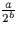, but cannot guarantee that (a,b) is a valid dyadic digit in its lowest term representation. Given a pair of integers (a,b) with the properties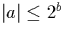and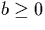, we show how to generate the dyadic digit (a',b') such that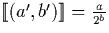.The operation is performed by examining a. If a is zero or odd, the dyadic digit may be obtained directly as follows:

If a is even and not equal to zero, we observe that (b>0) because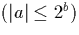, and so:

In this case we simply recursively call the function with the arguments (a/2,b-1) in this case until a is zero or odd, at which point we can return the dyadic digit.

Martin Escardo
5/11/2000